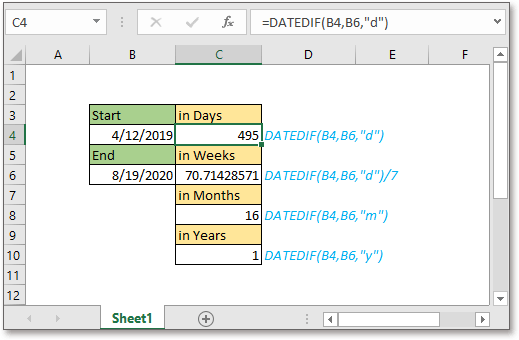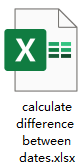Note: The other languages of the website are Google-translated. Back to English

## Excel Formula: Calculate Difference Between Two Dates in Years/Months/Weeks/DaysSometimes, in Excel worksheet, we need to calculate the difference between two dates for getting the durations. Here in this tutorial, it introduces the formulas on calculating difference between two dates in years, months, weeks, and days.Generic formula:

Calculate difference in days

 DATEDIF(start_date,end_date,"d")

Calculate difference in weeks

 DATEDIF(start_date,end_date,"d")/7

Calculate difference in months

 DATEDIF(start_date,end_date,"m")

Calculate difference in years

 DATEDIF(start_date,end_date,"y")

Arguments

 Start_datetime: The starting date in the date range. The start date must be smaller than the end date, or the formula will return the error result #NUM!. End_datetime: The ending date in the date range you want to calculate the date duration. The end date must be greater than the start date, otherwise the formula will return the error value #NUM!. D: Return difference in days. D/7:Return difference in weeks. 7 days a week, to return days firstly then divides 7 to get the number of weeks. As the formula returns value in date format, you need to format the result to general or number to get the number of weeks. M: Return difference in months. Y: Return difference in years.

How this formula work

Supposing you need to calculate the duration between the dates in cell B4 and B6, please use below formula

Calculate difference in days

 =DATEDIF(B4,B6,"d")

Calculate difference in weeks

 =DATEDIF(B4,B6,"d")/7

Calculate difference in months

 =DATEDIF(B4,B6,"m")

Calculate difference in years

 =DATEDIF(B4,B6,"y")

Press Enter key to get the result.

Tip: the formula to calculate difference in weeks will get the result in date format, just format the result cell as general or number as you need.Explanation

DATEDIF function: this function is used to calculate the difference between two dates in days, weeks, month, years, days ignoring years, or month ignoring years.

### The Best Office Productivity Tools

#### Kutools for Excel - Helps You To Stand Out From Crowd

Would you like to complete your daily work quickly and perfectly? Kutools for Excel brings 300 powerful advanced features (Combine workbooks, sum by color, split cell contents, convert date, and so on...) and save 80% time for you.

• Designed for 1500 work scenarios, helps you solve 80% Excel problems.
• Reduce thousands of keyboard and mouse clicks every day, relieve your tired eyes and hands.
• Become an Excel expert in 3 minutes. No longer need to remember any painful formulas and VBA codes.
• 30-day unlimited free trial. 60-day money back guarantee. Free upgrade and support for 2 years.#### Office Tab - Enable Tabbed Reading and Editing in Microsoft Office (include Excel)

• One second to switch between dozens of open documents!
• Reduce hundreds of mouse clicks for you every day, say goodbye to mouse hand.
• Increases your productivity by 50% when viewing and editing multiple documents.
• Brings Efficient Tabs to Office (include Excel), Just Like Chrome, Firefox, And New Internet Explorer.